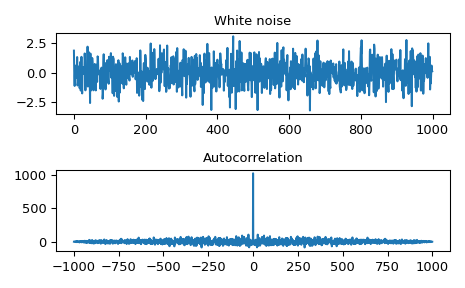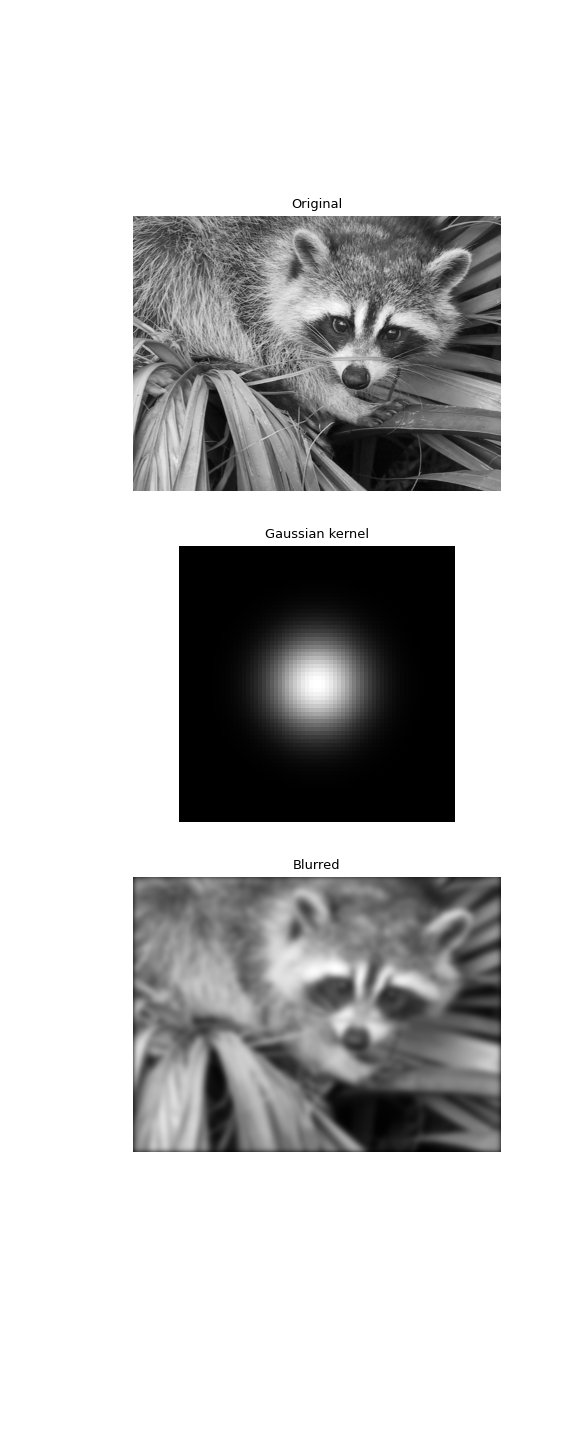# scipy.signal.fftconvolve¶

scipy.signal.fftconvolve(in1, in2, mode='full', axes=None)[source]

Convolve two N-dimensional arrays using FFT.

Convolve in1 and in2 using the fast Fourier transform method, with the output size determined by the mode argument.

This is generally much faster than `convolve` for large arrays (n > ~500), but can be slower when only a few output values are needed, and can only output float arrays (int or object array inputs will be cast to float).

As of v0.19, `convolve` automatically chooses this method or the direct method based on an estimation of which is faster.

Parameters
in1array_like

First input.

in2array_like

Second input. Should have the same number of dimensions as in1.

modestr {‘full’, ‘valid’, ‘same’}, optional

A string indicating the size of the output:

`full`

The output is the full discrete linear convolution of the inputs. (Default)

`valid`

The output consists only of those elements that do not rely on the zero-padding. In ‘valid’ mode, either in1 or in2 must be at least as large as the other in every dimension.

`same`

The output is the same size as in1, centered with respect to the ‘full’ output.

axesint or array_like of ints or None, optional

Axes over which to compute the convolution. The default is over all axes.

Returns
outarray

An N-dimensional array containing a subset of the discrete linear convolution of in1 with in2.

`convolve`

Uses the direct convolution or FFT convolution algorithm depending on which is faster.

`oaconvolve`

Uses the overlap-add method to do convolution, which is generally faster when the input arrays are large and significantly different in size.

Examples

Autocorrelation of white noise is an impulse.

```>>> from scipy import signal
>>> rng = np.random.default_rng()
>>> sig = rng.standard_normal(1000)
>>> autocorr = signal.fftconvolve(sig, sig[::-1], mode='full')
```
```>>> import matplotlib.pyplot as plt
>>> fig, (ax_orig, ax_mag) = plt.subplots(2, 1)
>>> ax_orig.plot(sig)
>>> ax_orig.set_title('White noise')
>>> ax_mag.plot(np.arange(-len(sig)+1,len(sig)), autocorr)
>>> ax_mag.set_title('Autocorrelation')
>>> fig.tight_layout()
>>> fig.show()
```

Gaussian blur implemented using FFT convolution. Notice the dark borders around the image, due to the zero-padding beyond its boundaries. The `convolve2d` function allows for other types of image boundaries, but is far slower.

```>>> from scipy import misc
>>> face = misc.face(gray=True)
>>> kernel = np.outer(signal.windows.gaussian(70, 8),
...                   signal.windows.gaussian(70, 8))
>>> blurred = signal.fftconvolve(face, kernel, mode='same')
```
```>>> fig, (ax_orig, ax_kernel, ax_blurred) = plt.subplots(3, 1,
...                                                      figsize=(6, 15))
>>> ax_orig.imshow(face, cmap='gray')
>>> ax_orig.set_title('Original')
>>> ax_orig.set_axis_off()
>>> ax_kernel.imshow(kernel, cmap='gray')
>>> ax_kernel.set_title('Gaussian kernel')
>>> ax_kernel.set_axis_off()
>>> ax_blurred.imshow(blurred, cmap='gray')
>>> ax_blurred.set_title('Blurred')
>>> ax_blurred.set_axis_off()
>>> fig.show()
```